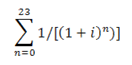# Calculating APR (Part 2)September 26, 2019

In Part 1 of our series on how to calculate APR, we discussed the mathematical procedure for deriving the APR from a payment flow. In that example, we had 24 even monthly payments of \$230 for a total repayment of \$5,520 on a loan of \$5,000. The procedure told us what APR would result in the \$520 of interest. The answer was 9.69%

Today, we are going to look at the effect of having an extra 19 days in the first period. In this example, we will assume a loan of \$6,000 with 36 even monthly payments of \$200 (\$7,200 total repayment; \$1,200 interest), but we will advance our money on February 10 (of a non leap year) and have our first payment due on April 1, with all subsequent payments on the first of the month.

So, why do the extra days in February matter? We are accruing extra days of interest and applying more of our first payment toward interest than we would if we did not have extra days, but this, by itself, does not cause the APR to go up. However, it does affects the payment stream and the total amount of interest accrued. If we work backward from the payment stream into the APR and use our previous assumptions that each payment period was of the same length, then the extra interest will result in and artificially high APR. We need to add a factor to the equation to account for these extra days, and if we do it right, it should be a factor that cancels out of the equation in a case where we do not have extra days. In other words, if we used the same set of equations and procedures in our previous example, they would have returned the same results.Where i is the periodic interest rate and t is the number of payments. The result of the above summation was then multiplied by the payment amount and divided by 1+i.

So, our extended equation looks like this: (Pmt X Summation)/(1+i)^t

Where the summation component is the equation shown above, and t = the number of unit periods per payment.

The component that we are going to add to the equation to account for the extra days will be a component we will designate as "f". This variable will be defined as a fraction of the number of extra days (in this case 19 days from February 10 through February 28, inclusive) over the standard number of days in the unit period. The unit period, in this case is months, and the standard number of days is 30 for a monthly unit period.

Here is the list of the number of days to use for the various unit periods:

 Weekly 7 Bi-Weekly 14 Semi-Monthly 15 Monthly 30 Bi-Monthly 60 Quarterly 90 Semi-Annually 180 Annually 360

So, in the case of our working example, f = 19/30. It is factored into the extended equation as follows:

(Pmt X Summation)/((1+fi)(1+i)^t)

The important thing to note is that when f = 0, as it did in our previous example (see part 1) the term of the equation 1+fi becomes 1+ 0 x i = 1. Having 1 as a factor in the denominator of the equation is equivalent to having that term of the equation removed entirely. So, we have met our goal of creating a general equation that handles the situation where we have an irregular first period, while still having the case of regular periods unchanged.

Now, let's complete our example.

Doing exactly the same procedure as in Part I, we set our trial APR (I) to 1 and i = I/12 = .000833333.

We take 1+i (1.000833333), and we raise it to each of the powers 0, 1, 2, 3, ....35. For each of our payments 1 through 36. We take the reciprocal of each of those 36 results and add them together and the resulting number is 35.48035.

We multiply by our payment (\$200) to get 7096.070678.

Next we take the two factors that need to be divided. 1+fi comes to 1.000527778 and (1+i)^t comes to 1.000833333 (there is one unit period per payment). Dividing the number above by the product of these factors we get 7086.422154. This is the value we call A'.

Next, we set I to 1.1% and run through the same procedure to get A'' = 7075.197593.

Then we use the interpolation equation (I = I(1) + 0.1 x [(A-A')/(A''-A')]) to derive I = 10.67897238.

And we have finished the first iteration. We use that value to begin the second iteration, running through the whole procedure again, and get I = 11.80439494

Using that value as the seed for the third iteration, we get I = 11.81651927

And using that value as the seed for the fourth iteration, we get I = 11.81650828

The government requirement is for two decimal place precision, and the example from The Federal Register Vol 76 # 246 pg 137 (example ii) gives the answer as 11.82%.

The Nortridge Loan System stops iterating when the values of the first four decimal places stop changing, and in this case, we are there without any further iterations. The value returned by the loan system would be 11.8165%.

As you can see, the procedure was mostly unchanged, but the extra 19 days of interest did have an impact on the portion of the equation that derived A' and A''. If we take the exact same procedure with all of the same numbers but with f = 0 (no long first period) we would expect to see a higher APR (same amount of interest crammed into less time means a higher rate). In fact, if I set f = 0 in this example, the final answer becomes: 12.2489%. The 19 days is in fact a significant factor.